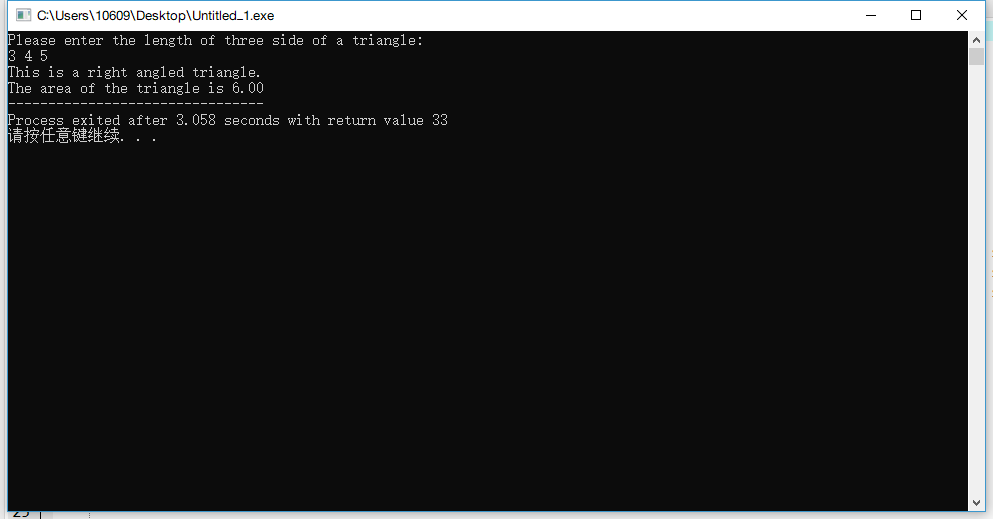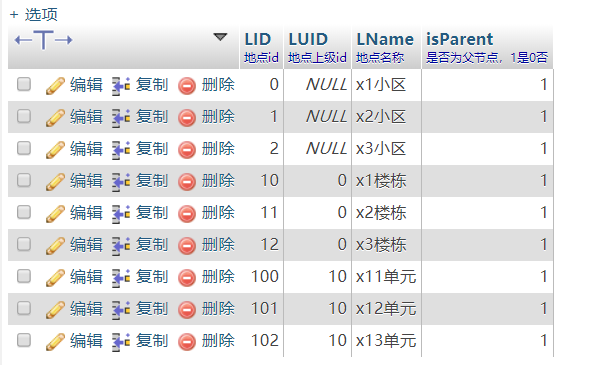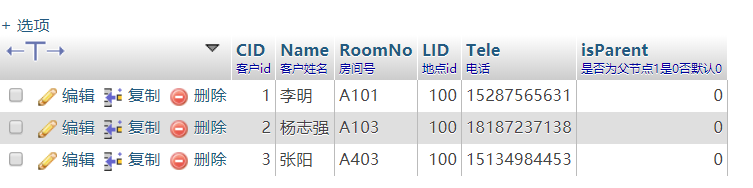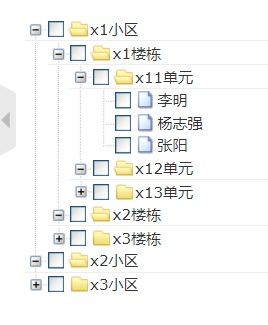• //我们将obj1初始化为一个Cube立方体，当然我们也可以初始化为其他的形状 GameObject obj1 = GameObject.CreatePrimitive(PrimitiveType.Cube); //设置物体的位置Vector3三个参数分别代表x,y,z的坐标数 obj1....

public class TestSphere : MonoBehaviour {

private Vector3 centerPos;    //圆的中心点
private float radius = 3;     //半径距离
private float angle = 0;      //偏移角度
public Transform father;
void Start()
{
CreateCircle();
}

// 随机分布在球型表面 注意两个物体会重叠

public void CreateSphere()
{
centerPos = transform.position;

for (int i = 0; i < 10; i++)
{
Vector3 p = Random.insideUnitSphere * radius;
Vector3 pos = p.normalized * 2;

GameObject obj1 = GameObject.CreatePrimitive(PrimitiveType.Sphere);
//设置物体的位置Vector3三个参数分别代表x,y,z的坐标数
obj1.transform.position = centerPos+pos;
obj1.transform.localScale = new Vector3(0.1f, 0.1f, 0.1f);
Debug.Log(p.magnitude);
}
}

// 随机生成在圆圈的圈子上
public void CreateCircle()
{
centerPos = transform.position;
for (int i = 0; i < 100; i++)
{
Vector2 p = Random.insideUnitSphere * radius;
Vector2 pos = p.normalized * 2;

GameObject obj1 = GameObject.CreatePrimitive(PrimitiveType.Sphere);
//设置物体的位置Vector3三个参数分别代表x,y,z的坐标数
obj1.transform.position = new Vector3(pos.x,pos.y, centerPos.z);
obj1.transform.localScale = new Vector3(0.1f, 0.1f, 0.1f);
Debug.Log(p.magnitude);
}
}

#region  按角度生成在圆点周围

// 按角度排列圆圈

public void CreateCubeAngle30()
{
centerPos = transform.position;
//20度生成一个圆
for (angle = 0; angle < 360; angle += 20)
{
//先解决你物体的位置的问题
// x = 原点x + 半径 * 邻边除以斜边的比例,   邻边除以斜边的比例 = cos(弧度) , 弧度 = 角度 *3.14f / 180f;
float x = centerPos.x + radius * Mathf.Cos(angle * 3.14f / 180f);
float y = centerPos.y + radius * Mathf.Sin(angle * 3.14f / 180f);
// 生成一个圆
GameObject obj1 = GameObject.CreatePrimitive(PrimitiveType.Sphere);
//设置物体的位置Vector3三个参数分别代表x,y,z的坐标数
obj1.transform.position = new Vector3(x, centerPos.z, y);
}
}

// 生成螺旋   //原理 += 半径
public void CreateMosquitoCoil()
{
centerPos = transform.position;
// 每隔30度就生成一个小方块
for (int i = 0; i < 120; angle += 18, radius += 0.2f, i++)
{
// 根据原点,角度,半径获取物体的位置.  x = 原点x + 半径 * 邻边除以斜边的比例
float x = centerPos.x + radius * Mathf.Cos(angle * 3.14f / 180f);
float y = centerPos.y + radius * Mathf.Sin(angle * 3.14f / 180f);
//我们将obj1初始化为一个Cube立方体，当然我们也可以初始化为其他的形状
GameObject obj1 = GameObject.CreatePrimitive(PrimitiveType.Cube);
//设置物体的位置Vector3三个参数分别代表x,y,z的坐标数
obj1.transform.position = new Vector3(x, centerPos.z, y);
}
}

}


展开全文unity 圆形
• 从键盘输入三个数字代表三条线段的长度（表示线段长度的变量用双精度类型），如果三条线段能形成三角形则输出它是钝角、直角还是锐角三角形以及该三角形的面积（面积要求小数点后保留两位小数），如果不能形成三角形...
来源：大工慕课 链接  作者：Caleb Sung
题目要求
从键盘输入三个数字代表三条线段的长度（表示线段长度的变量用双精度类型），如果三条线段能形成三角形则输出它是钝角、直角还是锐角三角形以及该三角形的面积（面积要求小数点后保留两位小数），如果不能形成三角形则输出“X.XX，X.XX，X.XX不能形成三角形。”（X.XX是输入的线段长度，要求小数点后保留两位小数。）
解答思路
可先找到最大的边，然后根据它的平方与其他两边的平方和判断是什么类型的三角型。
注意细节
import的头文件包括math.h，用于计算arccos和运用M_PI来表示π。 计算出的角度是弧度制，为方便理解比较需要转换成角度制。 输入数据的时候无需带小数点，数字之间可用空格隔开。在命令行（就是运行程序的黑框框）里输入数据的时候，数据之间的分隔符号取决于代码中scanf语句：
scanf("%d%d", &a, &b)       用空格隔开
scanf("%d,%d", &a, &b)      用逗号隔开
解答示范
下面这个代码是我一开始没看提示，直接算出三角形三个角度大小算出来的，和解答思路有一点区别，与之相匹配的代码在后面：
#include<stdio.h>
#include<math.h>

void main()
{
float side_1, side_2, side_3, angle_1, angle_2, angle_3, max=0.0, s=0.0;
printf("Please enter the length of three side of a triangle: \n");
scanf("%f%f%f", &side_1, &side_2, &side_3);

if((side_1 + side_2 > side_3) && (side_2 + side_3 > side_1) && (side_1 + side_3 > side_3))
{
angle_1 = 180.0 / M_PI * acos((side_2*side_2 + side_3*side_3 - side_1*side_1) / (2.0 * side_2 * side_3));
angle_2 = 180.0 / M_PI * acos((side_1*side_1 + side_3*side_3 - side_2*side_2) / (2.0 * side_1 * side_3));
angle_3 = 180.0 / M_PI * acos((side_1*side_1 + side_2*side_2 - side_3*side_3) / (2.0 * side_1 * side_2));

s = 0.5 * side_1 * side_2 * sin(angle_3 * M_PI / 180.0);

max = angle_1;
if(angle_2 > max){
max = angle_2;
}
if(angle_3 > max){
max = angle_3;
}

if(max > 90.0){
printf("This is an obtuse angled triangle.");
}
else if(max < 90.0){
printf("This is an acute triangle.");
}
else{
printf("This is a right angled triangle.");
}

printf("\nThe area of the triangle is %.2lf", s);
}
else{
printf("Sides with the length of %.2lf, %.2lf, %.2lf cannot not make up a triangle!", side_1, side_2, side_3);
}

}
下面这段代码是修改后的版本，运算效率较上一个有很大提高：
#include<stdio.h>
#include<math.h>

int main(){
double a, b, c, p, s, max, angle;

printf("请输入三角形三边边长: \n");
scanf("%lf%lf%lf", &a, &b, &c);

if (a+b>c && a+c>b && b+c>a){

max = a;
angle = acos((b*b+c*c-a*a)/(2*b*c))*180.0/M_PI;
if(max < b){
max = b;
angle = acos((a*a+c*c-b*b)/(2*a*c))*180.0/M_PI;
}
if(max < c){
max = c;
angle = acos((a*a+b*b-c*c)/(2*a*b))*180.0/M_PI;
}

if(angle > 90.0){
printf("这是个钝角三角形。");
}
else if(angle < 90.0){
printf("这是个锐角三角形。");
}
else{
printf("这是个直角三角形。");
}

p = 0.5 * (a + b + c);
s = sqrt(p * (p - a) * (p - b) * (p - c));
printf("这个三角形的面积为%.2lf。", s);
}

else{
printf("%.2lf, %.2lf, %.2lf三边不能组成三角形。");
}
}
运行效果展开全文• PAGE 计算机组成原理A 考作业一参考答案 一选择题 1机器数_中零的表示形式是唯一的 A原码 B补码 C移码 D反码 答案BC 2某计算机字长16位采用补码定点小数表示符号位为1位数值位为15位则可表示的最大正小数为_最小负...
• 计算机组成原理 A 考作业一参考答案 一选择题 1机器数_中零的表示形式是唯一的 A原码 B补码 C移码 D反码 答案BC 2某计算机字长 16 位采用补码定点小数表示符号位为 1 位数值位为 15 位则 可表示的最大正小数为_...
• 获取指定文件夹下的所有文件夹于文件形成树结构存库，使用递归调用的方式来实现； 一 大体思路 ​ 1.首先需要一个默认指定的文件夹地址 ​ 2.需要一个树vo类用来存放每个文件或文件夹的结构数据 ...
获取指定文件夹下的所有文件夹于文件形成树结构存库，使用递归调用的方式来实现；
######一 大体思路
​ 1.首先需要一个默认指定的文件夹地址
​ 2.需要一个树形vo类用来存放每个文件或文件夹的结构数据
​ 3.处理函数的参数设计，需要一个可操作路径，及一个父类id
​ 4.处理函数的思路：首先需要对传入进来的路径参数做判断，此路径是否存在，不错在抛异常结束程序运行；
​ 存在则继而判断此路径是一个文件夹，还是一个文件，若是一个文件执行存数据封装存库操作，return结束函数运行；
​ 若是一个文件夹也需要先进行数据封装存库，返回id做下一层子结构的父id，然后获取当前文件夹下面的所有文件夹及文件的全路径，循环调用当前函数，传入刚才存入的目录id，形成递归调用，直至函数结束运行；
二 代码实现
//我这里没有存库操作只是用来验证程序所以比较简单;
public class Tree {
private Integer id;
private String name;//文件夹或者文件名称
private String path;//全路径,或则部分路径,自己决定
private Integer parentId;//父节点id

public Tree() {
}

public Tree(Integer id, String name, String path,Integer parentId) {
this.id = id;
this.name = name;
this.path = path;
this.parentId = parentId;
}

public Integer getId() {
return id;
}

public void setId(Integer id) {
this.id = id;
}

public String getName() {
return name;
}

public void setName(String name) {
this.name = name;
}

public String getPath() {
return path;
}

public void setPath(String path) {
this.path = path;
}

public Integer getParentId() {
return parentId;
}

public void setParentId(Integer parentId) {
this.parentId = parentId;
}

@Override
public String toString() {
return "Tree{" +
"id=" + id +
", name='" + name + '\'' +
", path='" + path + '\'' +
", parentId=" + parentId +
'}';
}
}

//=====================================具体实现函数
public class FileUtil {
private static List<Tree> list = new ArrayList<>();//用来存放数据
private static Integer id = 0;//因为测试使用，当初主键id来用
public static void main(String[] args) {

String filepath="G:\\文件夹扫描";//默认路径，扫描此文件夹下面的所有文件
File file = new File(filepath);
int parentid = 0;  //初始化父节点id
try {
file(filepath,parentid);
} catch (FileNotFoundException e) {
e.printStackTrace();
}
for (int i = 0; i < list.size(); i++) {
Tree tree = list.get(i);
System.out.println("id:"+tree.getId()+"  parentId:"+tree.getParentId()+"=="+tree.getPath());
}
/*   String filepath="G:\\文件夹扫描";
File file = new File(filepath);
System.out.println(file);
System.out.println(file.exists());
System.out.println(file.isFile());
System.out.println(file.isDirectory());
System.out.println(file.getParent());
System.out.println(file.getName());
System.out.println(file.getPath());
System.out.println(file.getAbsoluteFile());
System.out.println(file.getAbsolutePath());
System.out.println(file.getAbsolutePath().replace("G:\\文件夹扫描",""));
System.out.println("-----------");
String[] list = file.list();
for (int i = 0;i<list.length;i++)
System.out.println(list[i]);*/
}

public static void file(String filepath,int parentid) throws FileNotFoundException {
File file = new File(filepath);
//1.判断文件
if(!file.exists()){
throw new FileNotFoundException("文件不存在");
}
//2.是文件该怎么执行
if(file.isFile()){
String name = file.getName();
String path = file.getAbsolutePath();
Tree tree = new Tree(id++,name,path,parentid);
return ;
}
//3.获取文件夹路径下面的所有文件递归调用；
if(file.isDirectory()){
String name = file.getName();
String path = file.getAbsolutePath();
Tree tree = new Tree(id++,name,path,parentid);
String[] list = file.list();
String parent = file.getParent();
for (int i = 0;i<list.length;i++){
String s = list[i];
String newFilePath = path+"\\"+s;//根据当前文件夹，拼接其下文文件形成新的路径
file(newFilePath,tree.getId());
}
}
}
}


展开全文递归 文件操作
• 项目目标：从MySQL数据库获取小区用户位置信息、用户信息利用ztree动态生成树菜单 mysql数据库表设计 小区地点表 小区用户表 控制器代码 /** * 获取小区用户位置信息\用户信息，并转换为json格式...
—搞了好久好久，在此记录一下这个这个胜利的时刻！！！！作为一个合格的程序员，任重而道远啊—

项目环境：thinkphp5+mysql+ztree  项目目标：从MySQL数据库获取小区用户位置信息、用户信息利用ztree动态生成树形菜单
mysql数据库表设计  小区地点表小区用户表控制器代码
/**
* 获取小区用户位置信息\用户信息，并转换为json格式，传给前端展示
*/
public function getLocationInfo(Request $request){ //获取ztree自带提交的参数， 若是页面加载时第一次请求， 该参数为空，$father_id = $request->param('id'); //dump($father_id);

//全局数据组
$data = array(); //当请求提交的参数id为空时， 只获取父菜单， if (!isset($father_id)) {//get all father menu
//dump("进来了，只获取父菜单");
$tempData = array(); //获取数据$tempData = LocationModel::all(['LUID'=>NULL]);

$a = array();$temp = array();

/*
* JSON数据封装时注意事项， 要求返回的json数据属性为父
* {"id":"52","name":"","pid":"null","isParent":true}
* 其中id为子节点id， pid为父节点id， name为节点名， isParent标明该节点是否属于父
* 节点， 这四个属性**必须**
*/

foreach ($tempData as$value) {
$temp['id'] =$value['LID'];
$temp['name'] =$value['LName'];
$temp['pid'] =$value['LUID'];
$temp['isParent'] =$value['isParent'];

//加了[],相当于把$a(一维数组)转变为二维数组(自己总结的~~)$a[] = $temp; unset($temp);
}
//将临时数组$a赋值给全局数组$data，作为返回
$data =$a;
unset($a); } else { //子节点被点击时，即从前端传来的$father_id不为空时

//获取父节点长度，用于判断是加载二三级目录还是加载客户名录列表（小区位置表与小区用户表不是一张表，需要关联查询）
$father_id_length = strlen($father_id);

//如果获取到的id长度小于3,只加载二级三级目录（二级目录楼栋，三级目录单元）
if($father_id_length < 3){ //dump('<<<<<3');$tempData = array();

$tempData = LocationModel::all(['LUID'=>$father_id]);

$a = array();$temp = array();

foreach ($tempData as$value) {
$temp['id'] =$value['LID'];
$temp['name'] =$value['LName'];
$temp['pid'] =$father_id;
$temp['isParent'] =$value['isParent'];
$a[] =$temp;
unset($temp); }$data = $a; unset($a);

//1、获取客户列表
//2、用取得的id(前端通过点击节点传来的父节点id)去客户表（customer）中查询是否存在该父节点下系列客户
//若存在则返回json数据，不存在不返回
//3、find()-查询单条数据，若查不到数据返回null
} else if(LocationModel::table('customer')->where('LID',$father_id)->find()){ //dump('>>>>>>>>3');$tempData1 = LocationModel::table('location loc, customer cus')
->where('loc.LID = cus.LID')
->field('cus.CID,cus.Name,cus.RoomNo,cus.LID,cus.isParent')
->order('cus.CID asc' )
->select();

$a1 = array();$temp1 = array();

foreach ($tempData1 as$value) {
$temp1['id'] =$value['CID'];
$temp1['name'] =$value['Name'];
$temp1['pid'] =$value['LID'];
$temp1['isParent'] =$value['isParent'];
$a1[] =$temp1;
unset($temp1); }$data = $a1; unset($a1);
}
}

//json数据返回
//将数组转化为json返回，thinkPHP5
return json_encode($data); } 前端htmL代码 <!--引入zTree css样式文件--> {load href="__STATIC__/lib/zTree/v3/css/zTreeStyle/zTreeStyle.css" /} {/block} <!-- ztree容器 --> <ul id="mytree" class="ztree"></ul> <!--引入zTree js文件--> <script type="text/javascript" src="__STATIC__/lib/zTree/v3/js/jquery.ztree.all-3.5.min.js"></script> <script type="text/javascript"> var setting = { //设置数据格式 data: { simpleData: { enable: true, idKey: "id", pIdKey: "pId", rootPId: 0, } }, //可勾选 check: { enable: true, chkStyle: "checkbox", }, async: { enable: true, dataType: "json", url: "getLocationInfo", autoParam: ["id"], type:'post', }, // 回调函数 callback : { beforeAsync: zTreeBeforeAsync, onAsyncSuccess: zTreeOnAsyncSuccess, onAsyncError: zTreeOnAsyncError, }, }; //用于捕获异步加载之前的事件回调函数，zTree 根据返回值确定是否允许进行异步加载 function zTreeBeforeAsync(treeId, treeNode) { //alert("这是异步加载之前的事件回调函数"); }; //异步请求成功的回掉函数 //treeId-mytree function zTreeOnAsyncSuccess(event, treeId, treeNode, msg) { //alert(msg); }; //异步请求失败的回调函数 function zTreeOnAsyncError(event, treeId, treeNode, XMLHttpRequest, textStatus, errorThrown) { alert('请求失败！'); alert(XMLHttpRequest); };$(function() {
$.fn.zTree.init($("#mytree"), setting);
});
</script>

效果图完结！！！！！！！！！！  ———————-重新更新过，现在更完整!—————————-
展开全文ztree mysql thinkphp5 ajax
• 圆上任选三点组成三角形，这个三角形是锐角、钝角和直角三角形的概率分别是多少？ 问题可以等价于： 任取三角形，圆心落在三角形内、外、边上的概率各是多少 这三种情况分别对应锐角、钝角、直角。 然后这就...三角形
• 第一步，获得树数据 对于渲染成一个树菜单，也就是我们平时见到的多级菜单，我们首先要将数据处理成树结构。这里笔墨不多写了，假设我们拿到的demoData就已经是的了。...这里同时要注意vue是使用props从父前端 vue-js
• 获取键盘输入的三角形三边,判断是否可以组成三角形,如果能组成三角形,进一步判断是什么三角形(普通、等腰、等边、直角) 分析： 看到这个题目，我们首先应该想到的是用if 条件语句，在分析形成三角形的条件是什么？...python 软件测试 经验分享
• 输入三个数,表示三角形三边长度,判断它是一个什么类型的三角形 */ 判断三角形成立的条件 两边之和大于第三边. if...else语句的运用 逻辑运算符&&和||的使用 &&运算符用于两个或者多个...C语言 判断三角形
• def is_triangle(a, b, c): if (a<=0 or b<=0 or c<=0): return -1 elif((a+b)>c and (a+c)>b and (b+c)>a): return 1 else: return 0python
• Eddy对这道题目百思不得其解，想不通用什么方法来解决，因此他找到了聪明的你，请你帮他解决这个题目。   Input 输入数据包含多测试用例，每个测试用例的第一行包含一个整数n，表示一共有n个互不相同的点，接下来...
• 将两根矩形钢管直接焊接形成新型T钢管混凝土组合构件，进行了8共16个T钢管混凝土组合构件的抗弯试验，分析了含钢率、剪跨比、截面尺寸等参数对新型T钢管混凝土组合构件抗弯性能的影响，比较了新型T钢管混...
• 控件为目录状字符串：树控件列字符串可形成树型目录状，以后各列为一般字符串，可以用来添加各注说明等；树控件的每一行是一个项，拖动项可以改变项所在的目录，如图1所示。 　右键单击树控件，在弹出的...
• 在最近的论文arXiv：1606.02921中，在超空间中构造了6D N = 1 0 $$\ mathcal {N} = \ left（1,0...并重构其大部分 通过提升N = 1 0$$ \ mathcal {N} = \ left（1,0 \ right）不变量的某种线性组合来形成Bosonic项。
• 本文对出露于吉林省中部地区的小蜂蜜顶子标准剖面的流纹岩(样品号:JH21-1)和非标准剖面小蜂蜜顶子的安山岩(样品号:JX1-1)进行了锆石LA-ICP-MS U-Pb年代学以及Hf同位素组成研究。2个样品中的锆石均呈自―半自...
•技术
• 一条长度为l的线段，随机在其上选2个点，将线段分为3段，问这3个子段能组成一个三角形的概率是多少？   <br />1/4。 假设我们选择的两个点的坐标是x和y（先假设x ）：  那么由三角形...
• 先画一个由N个点和N条边组成的圈，称这个圈为“核心圈”，然后对于核心圈的每一条边，将其扩展成一个五角圈，也就是增加3个点和4条边，这样就形成了一个由N个五角形组成的五角圈，简称N五角圈。 分析：每一个...
• 问题1：遍历所有可能的3个点 1 2 3  for(int i = 0; i &lt; n; i++)  for(int j = i + 1; j &... 问题2：判断3个点是否能组成三角形 1 2 ...
• 用户输入三个整数，判断这三个整数能否构成... if (a+b>c && a+c>b && b+c>a) { printf("以%d,%d,%d为三条边能够组成三角形\n",a,b,c); } else { printf("以%d,%d,%d为三条边不能组成三角形\n",a,b,c); } return 0; }c语言 算法
• Unity3D中Mesh的基本单位是三角形，而圆形就是由许许多多的三角形组成的。我们绘制N个等腰三角形，把它们拼起来，就形成了一个圆。 而圆环则是由N个等腰梯形组成，而每个梯形则是由两个三角形组成Mesh Unity3D 圆形
• 需要实现 UI 给出的下面两种图中带...将气泡分解可知，气泡由 正/倒三角形 和 圆角长方形 组成，于是可以通过组合来形成三角形气泡的效果。参考 这篇博文 绘制三角形。正三角形的 triangle_up.xml 如下： <layer-listandroid shape 三角形
• 在Sunny软件公司的内部办公系统Sunny OA系统中，有一个与公司组织结构对应的树菜单，行政人员可以给各级单位下发通知，这些单位可以是总公司的一个部门，也可以是一个分公司，还可以是分公司的一个部门。...组合模式
• 读书破万卷 下笔如有神 计算机组成原理 A 形成性考核作业三参考答案 一选择题 1下列部件设备中存取速度最快的是 _ 答 B A 光盘存储器 B CPU 的寄存器 C软盘存储器 D硬盘存储器 2某 SRAM 芯片其容量为 1K8 位加上电源...
• 电大计算机组成原理形成性考评册答案 计算机组成原理A 考作业一? 一选择题 1机器数_中零表示形式是唯一B 2某计算机字长16位采取补码定点小数表示符号位为1位数值位为15位则可表示最大正小数为_最小负小数为_C 3...
• 从下面的三角形的顶端开始，向下面一行的相邻数字移动，从顶端到底端的最大总和为23. 也就是 3 + 7 + 4 + 9 = 23. ...很大程度当我们看到这样问题时，会马上形成一条简单思路，那就是：记录从顶点到算法
• 2013秋电大计算机组成原理形成性考核册答案 计算机组成原理A 考作业一 一选择题 1机器数_中零的表示形式是唯一的B 2某计算机字长16位采用补码定点小数表示符号位为1位数值位为15位则可表示的最大 正小数为_最小负......